# Gear Torque Basics

Hi everyone

I'm trying to write a computer simulation of meshing gears (actually its for a videogame), but my understanding of gears and torque is sadly lacking and I'm having trouble finding the answers to what I think are probably very basic questions. I apologise if I've posted this in the wrong place, by the way - it's my first time here.

Firstly, let's suppose there are 2 connected gears of equal size, A and B. Motor M applies a torque to gear A, and we'll assume friction is negligable: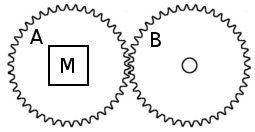Am I correct in thinking that both gears are capable of delivering the same amount of torque that the motor is applying? At the same time?

Then we have the case of different gear ratios. Lets say that gear B has twice as many teeth as gear A: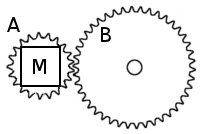I understand that the gear ratio means that gear B rotates at half the speed of gear A, but delivers twice the torque. Although I am not entirely sure why.

For a sequence of gears like this: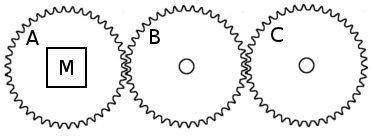I can understand each gear delivering the same torque because I can sort of imagine that gear A applies all its force to gear B, which applies all its force to gear C.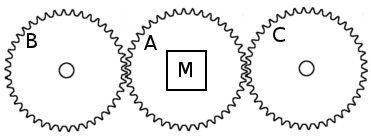Surely here gears B and C are only receiving half of A's force each. Does this mean B and C deliver half as much torque as A?

And following that line of reasoning: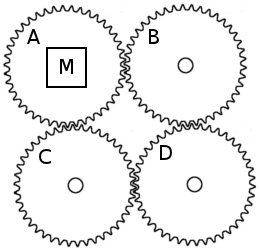Do gears B and C deliver half as much torque each as A or D? In other words does D deliver the same amount of torque as A, but B and C don't?

And with 2 motors, now, both applying torque in the same direction: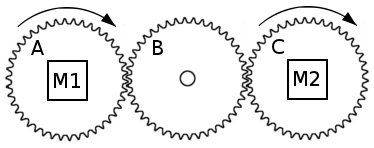In this configuration would B deliver the torque of A and C combined?

I imagine I have misunderstood something fundamental here. If anyone could steer my thinking in the right direction I'd be very grateful.

rcgldr
Homework Helper
Unless there is an external torque on the driven gears to oppose the torque from the motor, then the torque just ends up accelerating all the gears, based on the total angular inertia.

If more than one gear has an external torque, then the torque at each gear with an external torque is less than if only one gear had an external torque. The arrangement of the gears doesn't matter, except for the direction of the torque. The relative size of the gears multiplies torque and divides angular veloctiy or vice versa.

Frimkron
You are right in all the cases. You have no misconception so far.
In the second case where you don't understand why the second gear would deliver double torque, the reason is that its radius is double that of the smaller gear.
Have a nice time.
:)

You can post such posts in the section of Mechanical Engineering.

Thanks rcgldr, jack action and R Power.
rcgldr said:
Unless there is an external torque on the driven gears to oppose the torque from the motor, then the torque just ends up accelerating all the gears, based on the total angular inertia.
And I guess the acceleration of the gears would be calculated as (total motor torque - total opposing torque) / total moment of inertia?
jack action said:
power in = power out

the power in is the sum of the power from all your motors. The torque (N.m) at each gear will be equal to the net power (W) applied on that gear divided by its rpm (rad/s).
Ok, I think I understand. So let's say I have multiple motors and multiple loads. The total power in will be the sum of the power from the motors, and this will equal the total power out. But will the power out be distributed equally to each of the load gears, or is there more to it than this?

And I have a follow-up question concerning torque translating to linear force. Let's say there's load in the form of a hammer H connected to gear B, and this strikes another object: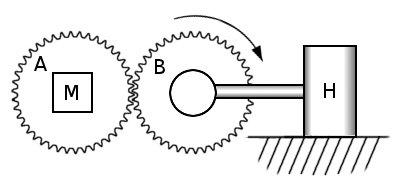At the point of impact, I need to know the momentum of the hammer head in order to resolve the collision. I presume the linear velocity of the hammer head could be calculated by $$v = r\omega$$, where $$r$$ is the length of the hammer and $$\omega$$ is the angular velocity of gear B. But what about the mass? Would this include the mass of the gears?

Then let's say the hammer remains in contact with the object. As far as I understand, the contact force would calculated by $$F = \frac{\tau}{r}$$ where $$\tau$$ is the torque at gear B. Is this correct?

At the point of impact, I need to know the momentum of the hammer head in order to resolve the collision. I presume the linear velocity of the hammer head could be calculated by , where is the length of the hammer and is the angular velocity of gear B. But what about the mass? Would this include the mass of the gears?

Then let's say the hammer remains in contact with the object. As far as I understand, the contact force would calculated by where is the torque at gear B. Is this correct?
No, in case of momentum of hammer it won't include mass of gears. You only need to know mass of the hammer itself.
And for contact force I think you are correct but I'm not that sure here!!!

R Power said:
No, in case of momentum of hammer it won't include mass of gears. You only need to know mass of the hammer itself.
The reason I ask is because surely to alter the velocity of the hammer a force must be applied which accelerates the entire gear system.

jack action
Gold Member
Ok, I think I understand. So let's say I have multiple motors and multiple loads. The total power in will be the sum of the power from the motors, and this will equal the total power out. But will the power out be distributed equally to each of the load gears, or is there more to it than this?

The power goes wherever it needs to go. If the resistance increases at gear A (or B or C), total power generated by the motors must increase an equal amount, doesn't matter which motor it comes from. The net power on each gear is the power from the motor acting on that gear (if any) minus the power from the resistance acting on that gear (if any). The difference must come from (or go to) the other gears connected to it. If there is no other power source (or resistance) to balance the power equation, the whole system will accelerate (or decelerate) with that power difference (considering the inertia, angular velocity and torque for all gears and the systems connected to them).

Then let's say the hammer remains in contact with the object. As far as I understand, the contact force would calculated by $$F = \frac{\tau}{r}$$ where $$\tau$$ is the torque at gear B. Is this correct?

This is correct. In that case there is no motion so power is zero. So the proper balance equation is the force balance equation: FA = FB, where FA is the force acting on the teeth at gear A and FB is the opposing force acting on the teeth at gear B. Each force multiply by its respective radius gives the gear torque. TA being the torque produced by the motor and TB being the torque applied by your hammer (as you calculated it).

And I have a follow-up question concerning torque translating to linear force. Let's say there's load in the form of a hammer H connected to gear B, and this strikes another object:At the point of impact, I need to know the momentum of the hammer head in order to resolve the collision. I presume the linear velocity of the hammer head could be calculated by $$v = r\omega$$, where $$r$$ is the length of the hammer and $$\omega$$ is the angular velocity of gear B. But what about the mass? Would this include the mass of the gears?

The problem here is more complex as the impact force depends a lot on material property (how they will deform under impact). This deformation will give you a distance "traveled" by the hammer to achieve a complete stop and, knowing the initial velocity (as you stated, $$v = r\omega$$), will give you the deceleration rate of the hammer AND gear set. So inertia of the gears are also important.

Then let's say the hammer remains in contact with the object. As far as I understand, the contact force would calculated by where is the torque at gear B. Is this correct?
I think the force applied by hammer will not come alone from the torque at gear B. Here gravity will also play it's role. If W is weight of hammer then torque at B will be increased by an amount= (W x lenght of hammer).

I think the force applied by hammer will not come alone from the torque at gear B. Here gravity will also play it's role.
You're correct of course, but for my purposes I can disregard gravity.

I think it's going to make more sense for me to think in terms of forces rather than power, because as far as I understand the power balance equation only applies where the system is in equilibrium and the gears are rotating at a fixed rate, whereas I need to model the acceleration of the gear system.

Regarding the acceleration of the gears, I'm still unsure as to how to calculate this. For example, consider this configuration where 2 motors attached to differently-sized gears both apply torque to a system with no load: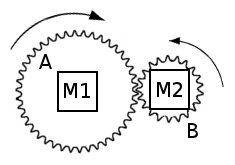The motors will accelerate the gears, but how is this acceleration calculated? I know that for acceleration $$a$$ torque $$\tau$$ and moment of ineria $$I$$: $$a = \frac{\tau}{I}$$. But if I take the total torque and total MoI, how does an acceleration in rad/sec2 relate to differently-sized gears? The gears have to rotate at different velocities because of their relative sizes!

jack action said:
So the proper balance equation is the force balance equation: FA = FB, where FA is the force acting on the teeth at gear A and FB is the opposing force acting on the teeth at gear B. Each force multiply by its respective radius gives the gear torque. TA being the torque produced by the motor and TB being the torque applied by your hammer (as you calculated it).

This makes sense. So, where are the forces acting when we have more than one motor and more than one hammer? For example: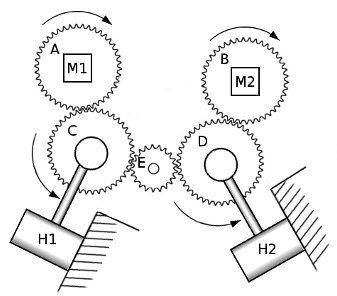Obviously here motor M1 applies a force to the object H1 is in contact with, via gears A and C, and motor M2 applies a force to the object H2 is in contact with, via gears B and D. But does M1 also affect H2, and M2 affect H1 via gear E? If so, how much force does each motor apply to each hammer? Does half of gear C's torque apply to H1 and half to gear E? And would M1 apply a force to M2 and vice versa?

If M1 does not affect H2 and M2 does not affect H1, then what about if these gears are added: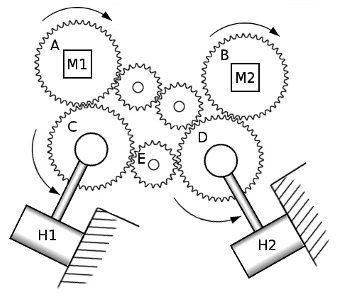I think I'm having trouble tracing where forces are acting. It seems to me that the arrangement of the gears would be important in these cases, but I could be wrong.

jack action said:
The problem here is more complex as the impact force depends a lot on material property (how they will deform under impact). This deformation will give you a distance "traveled" by the hammer to achieve a complete stop and, knowing the initial velocity (as you stated, $$v = r\omega$$ ), will give you the deceleration rate of the hammer AND gear set. So inertia of the gears are also important.

I would model the impact as a rigid body collision, disregarding deformation completely and assuming an instantaneous change in the velocity of both objects at the time of collision, caused by some impulse J (more info here), so I'm concerned only with the momentum of the colliding objects. But I wonder whether it's the exchange of angular or linear momentum that's important here. Obviously the hammer can only rotate, whereas the object it strikes can move linearly.

jack action
Gold Member
Regarding the acceleration of the gears, I'm still unsure as to how to calculate this. For example, consider this configuration where 2 motors attached to differently-sized gears both apply torque to a system with no load:The motors will accelerate the gears, but how is this acceleration calculated? I know that for acceleration $$a$$ torque $$\tau$$ and moment of ineria $$I$$: $$a = \frac{\tau}{I}$$. But if I take the total torque and total MoI, how does an acceleration in rad/sec2 relate to differently-sized gears? The gears have to rotate at different velocities because of their relative sizes!

At any moment, this relation will be true (It uses power, whether you like it or not):

$$\frac{P_{M1}}{\omega_{A}} + \frac{P_{M2}}{\omega_{B}} = I_{A}\alpha_{A} + I_{B}\alpha_{B}$$

where angular velocities and accelerations of the gears are related to each other by the gear ratio (because at the contact point, both gears have the same linear velocity and acceleration).

So, where are the forces acting when we have more than one motor and more than one hammer? For example:Obviously here motor M1 applies a force to the object H1 is in contact with, via gears A and C, and motor M2 applies a force to the object H2 is in contact with, via gears B and D. But does M1 also affect H2, and M2 affect H1 via gear E? If so, how much force does each motor apply to each hammer? Does half of gear C's torque apply to H1 and half to gear E? And would M1 apply a force to M2 and vice versa?

If M1 does not affect H2 and M2 does not affect H1, then what about if these gears are added:I think I'm having trouble tracing where forces are acting. It seems to me that the arrangement of the gears would be important in these cases, but I could be wrong.

The important relationship to understand with gears is that at the contact point between 2 gears the forces acting on the tooth of each gear are the same and the linear velocities of each tooth are also the same. From there, you can find torque and angular velocity for each gear.

The problem with the gear set presented above is that they contain redundant gears, meaning that power transmission can take one path or another. When there is symmetry, you can divide the force equally between the paths. For example, in this case:

only one idler gear is necessary. The other one is only there to "pull" on the first one such that it doesn't fall. But if there was a shaft welded to cylinder block, the second gear would be unnecessary.

In real life, in such system, the power can be transmitted through one path only due to manufacturing tolerances; the other gears would become simple idlers because they would be "loose". So as far as how much torque goes through which gear set, all answers can be true in a redundant mechanism.

For example, to be stable a table needs only 3 legs; the fourth one is redundant and may have no weight at all resting on it. If tolerances are good and the floor is perfectly flat, you can assume that each leg supports 1 quarter of the weight because of symmetry. But add a fifth leg randomly and your guess becomes as good as mine.

But I wonder whether it's the exchange of angular or linear momentum that's important here. Obviously the hammer can only rotate, whereas the object it strikes can move linearly.

It's both, see equation (3):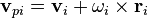If the object is 100% linear or 100% angular, then one of the components will be zero.

Last edited by a moderator:
In the picture of the V8 engine you posted my guess about using 2 equal sized gears is that the purpose is to enhance the engine life. That is one gear would wear out too quickly. Auto manufactures do not include unnecessary parts.

At any moment, this relation will be true (It uses power, whether you like it or not):

$$\frac{P_{M1}}{\omega_{A}} + \frac{P_{M2}}{\omega_{B}} = I_{A}\alpha_{A} + I_{B}\alpha_{B}$$

Do motors generally have a fixed power output then, rather than a fixed torque? Would it be unrealistic to model my motors as having a fixed torque they apply? If a motor's power is constant, wouldn't this mean the torque approaches infinity at low angular velocity?

So anyway, correct me if I'm wrong but I think this is how I could calculate the acceleration for any given gear set: In the diagram above with 7 gears, let's say the hammers haven't hit yet and the motors are still accelerating the gear system. I know the power and angular velocity of the motors and therefore the combined input torque, $$\tau_{total}$$. I know the MoI of each large gear to be $$I$$ and $$\frac{1}{4}I$$ for each smaller gear. I know the diameter of the smaller gears to be half the diameter of the larger gears and therefore that if larger gears accelerate at a rate of $$\alpha$$, the smaller gears will accelerate at $$2\alpha$$. So then the following would hold true:

$$\tau_{total} = I\alpha + I\alpha + I\alpha + I\alpha + \frac{1}{4}I2\alpha + \frac{1}{4}I2\alpha + \frac{1}{4}I2\alpha$$

giving $$\alpha = \frac{\tau_{total}}{5\frac{1}{2}I}$$ from which I can calculate the acceleration of each gear. Is this correct?

jack action said:
The problem with the gear set presented above is that they contain redundant gears, meaning that power transmission can take one path or another. When there is symmetry, you can divide the force equally between the paths.
jack action said:
In real life, in such system, the power can be transmitted through one path only due to manufacturing tolerances; the other gears would become simple idlers because they would be "loose". So as far as how much torque goes through which gear set, all answers can be true in a redundant mechanism.

So assuming we have an ideal, perfectly manufactured gear set, the force each gear delivers to connected gears would be split equally? So in the 7-gear diagram, the torque at gear A, $$\tau_{A}$$ would translate to contact forces with C and the smaller gear, each of $$\frac{1}{2}\frac{\tau_{A}}{r}$$. Tracing the forces from A and B around all the paths, I end up with $$\frac{1}{4}\frac{\tau_{A}}{r} + \frac{2}{3}\frac{\tau_{B}}{r}$$ at H1 and $$\frac{3}{4}\frac{\tau_{A}}{r} + \frac{1}{3}\frac{\tau_{B}}{r}$$ at H2. Have I calculated that correctly?

rcgldr
Homework Helper
Do motors generally have a fixed power output then, rather than a fixed torque?
Engine (fuel based) have a torque versus rpm graph that is curved with a peak somewhere between minimum and maximum rpms. Motors (electrical) have a toque versus rpm curve that closely approximates a line with negative slope. In the case of an ideal DC motor, peak torque occurs at 0 rpm, peak power occurs at 1/2 of the maximum rpm, and there's zero torque at maximum rpm.

jack action
Gold Member
Do motors generally have a fixed power output then, rather than a fixed torque? Would it be unrealistic to model my motors as having a fixed torque they apply?

Electric motors are essentially constant power motor (fixed power rating). Hydraulic, pneumatic and internal combustion engine are essentially constant torque motor (fixed pressure acting on a cylinder). But there's usually always a variation of torque with rpm.

If a motor's power is constant, wouldn't this mean the torque approaches infinity at low angular velocity?

Yes it does. Although not an expert on the subject, usually there is some maximum torque that can be applied to an electric motor and the rest of the power is converted to heat. If this condition (rpm=0) is maintained too long, the motor will destroy itself by overheating.

So anyway, correct me if I'm wrong but I think this is how I could calculate the acceleration for any given gear set: In the diagram above with 7 gears, let's say the hammers haven't hit yet and the motors are still accelerating the gear system. I know the power and angular velocity of the motors and therefore the combined input torque, $$\tau_{total}$$. I know the MoI of each large gear to be $$I$$ and $$\frac{1}{4}I$$ for each smaller gear. I know the diameter of the smaller gears to be half the diameter of the larger gears and therefore that if larger gears accelerate at a rate of $$\alpha$$, the smaller gears will accelerate at $$2\alpha$$. So then the following would hold true:

$$\tau_{total} = I\alpha + I\alpha + I\alpha + I\alpha + \frac{1}{4}I2\alpha + \frac{1}{4}I2\alpha + \frac{1}{4}I2\alpha$$

giving $$\alpha = \frac{\tau_{total}}{5\frac{1}{2}I}$$ from which I can calculate the acceleration of each gear. Is this correct?

So assuming we have an ideal, perfectly manufactured gear set, the force each gear delivers to connected gears would be split equally? So in the 7-gear diagram, the torque at gear A, $$\tau_{A}$$ would translate to contact forces with C and the smaller gear, each of $$\frac{1}{2}\frac{\tau_{A}}{r}$$. Tracing the forces from A and B around all the paths, I end up with $$\frac{1}{4}\frac{\tau_{A}}{r} + \frac{2}{3}\frac{\tau_{B}}{r}$$ at H1 and $$\frac{3}{4}\frac{\tau_{A}}{r} + \frac{1}{3}\frac{\tau_{B}}{r}$$ at H2. Have I calculated that correctly?

For every contact between 2 gears (say gear 1 & 2), you will have 2 equations, based on force and acceleration at the contact point:

$$\frac{\tau_{1} - I_{1} \alpha_{1}}{r_{1}} = - \frac{\tau_{2} - I_{2} \alpha_{2}}{r_{2}}$$

$$\alpha_{1} r_{1} = - \alpha_{2} r_{2}$$

The negative sign keeps track of the rotation inversion.

For example, if you know $$\tau_{1}$$ because a motor is connected to it and nothing is connected to gear 2 ($$\tau_{2} = 0$$), then the only two unknowns are $$\alpha_{1}$$ and $$\alpha_{2}$$.

If you have a third gear connected to, say, gear 2, then you have 2 other equations:

$$\frac{\tau_{3} - I_{3} \alpha_{3}}{r_{3}} = - \frac{\tau_{2} - I_{2} \alpha_{2}}{r_{2}}$$

$$\alpha_{3} r_{3} = - \alpha_{2} r_{2}$$

So now you have 4 equations and you can solve for 4 unknowns ($$\alpha_{1}$$, $$\alpha_{2}$$, $$\alpha_{3}$$ and $$\tau_{2}$$; $$\tau_{3} = 0$$). Adding gears, will add equations. For your 5-gear arrangement, you should have a system of 8 equations (4 contact points), so 8 unknowns to solve. For your 7-gear arrangement, you should have a system of 14 equations. But you will find out that unknowns will not match (because of the redundant gear set). So you will need to assume extra relationships (hence extra equations) to solve the problem such as the one you made (force split equally between all contact points on a gear).

I think I got it right.

For every contact between 2 gears (say gear 1 & 2), you will have 2 equations, based on force and acceleration at the contact point:

$$\frac{\tau_{1} - I_{1} \alpha_{1}}{r_{1}} = - \frac{\tau_{2} - I_{2} \alpha_{2}}{r_{2}}$$

$$\alpha_{1} r_{1} = - \alpha_{2} r_{2}$$

The negative sign keeps track of the rotation inversion.
The first equation has me a little confused. Why is it $$\tau-I\alpha$$, exactly? What does this term represent? For a single gear, $$\tau=I\alpha$$, right? So if a single gear was in contact with something, the contact force would be $$\frac{I\alpha-I\alpha}{r} = 0$$??

For example, if you know $$\tau_{1}$$ because a motor is connected to it and nothing is connected to gear 2 ($$\tau_{2} = 0$$), then the only two unknowns are $$\alpha_{1}$$ and $$\alpha_{2}$$.

If you have a third gear connected to, say, gear 2, then you have 2 other equations:

$$\frac{\tau_{3} - I_{3} \alpha_{3}}{r_{3}} = - \frac{\tau_{2} - I_{2} \alpha_{2}}{r_{2}}$$

$$\alpha_{3} r_{3} = - \alpha_{2} r_{2}$$

So now you have 4 equations and you can solve for 4 unknowns ($$\alpha_{1}$$, $$\alpha_{2}$$, $$\alpha_{3}$$ and $$\tau_{2}$$; $$\tau_{3} = 0$$).
Let's say there are 3 gears connected in a line. Maybe I've misunderstood something here (or my algebra's gone wrong), but given the first 2 equations, I get:
$$\alpha_{1} = \frac{r_{2}\tau_{1} + r_{1}\tau_{2}}{r_{2}I_{1} - \frac{r_{1}}{r_{2}}r_{1}I_{2}}$$
which would allow me to calculate the acceleration of gears 2 and 3, given that $$\alpha_{2} = -\frac{r_{1}}{r_{2}}\alpha_{1}$$ and $$\alpha_{3} = -\frac{r_{2}}{r_{3}}\alpha_{2}$$. But this seems wrong, if the acceleration of the gear system is supposed to depend on the total moment of inertia of all three gears.

So you will need to assume extra relationships (hence extra equations) to solve the problem such as the one you made (force split equally between all contact points on a gear).
Regarding the force splitting: so I could assume that forces split equally to connected gears. But in the 5-gear setup above at gear C, some of the force from gear A is going to go to gear E and the rest is going to go to the object hammer H1 is in contact with. Would it be realistic to assume this would be an equal split too, or in this case would it be the torque that is split equally? Intuition makes me think that the force on gear E would be greater than the force at H1. Plus, (more scientifically) the lever principle is at work here isn't it, so the force in doesn't necessarily equal the force out.

Thanks for continuing to help me out jack action and rcgldr.

jack action
Gold Member
$$\tau$$ is the torque applied to the gear shaft; either from a motor or some resistance; even friction. $$I \alpha$$ is the «inertia torque» that is not available to the other gears.

First, I realized that I've put an extra minus sign, the proper equations would be:

$$\frac{\tau_{1} - I_{1} \alpha_{1}}{r_{1}} = \frac{\tau_{2} - I_{2} \alpha_{2}}{r_{2}}$$

$$\alpha_{1} r_{1} = - \alpha_{2} r_{2}$$

If you solved those equations you get:

$$\tau_{1} - \left( \frac{r_{1}}{r_{2}} \right) \tau_{2} = \left[ I_{1} + \left( \frac{r_{1}}{r_{2}} \right) ^{2} I_{2} \right] \alpha_{1}$$

Or:

$$\tau_{eq} = I_{eq} \alpha_{1}$$

Where $$\tau_{eq}$$ and $$I_{eq}$$ are the equivalent torque and inertia of the 2 gears that need to be accelerated, based on the acceleration of gear 1.

If you assume that a motor is on gear 1 and nothing on gear 2, then $$\tau_{1}$$ is the torque of the motor and $$\tau_{2} = 0$$ since there is no exterior input or output torque. So:

$$\tau_{1} = \left[ I_{1} + \left( \frac{r_{1}}{r_{2}} \right) ^{2} I_{2} \right] \alpha_{1}$$

If there was a torque applied at gear 2 then it would be added to (or subtracted from) the torque of gear 1. For example if we assume that clockwise direction is positive and that $$\tau_{1}$$ is positive, then a motor putting a counterclockwise torque (negative) on gear 2 would increase the total torque. If it was a clockwise torque (positive), it would work against the motor at gear 1, hence the subtraction.

If $$\tau_{eq}$$ is zero, then $$\alpha_{1}$$ must be zero, meaning the gear set is turning at a constant rpm.

If you have more gears, you will still end up with an equation of the form $$\tau_{eq} = I_{eq} \alpha_{1}$$. For the 3-gear example you did not solve the equation with $$\tau_{2}$$ and $$\tau_{3}$$ which will give $$\tau_{2}$$ as a function of $$\alpha_{1}$$ (since $$\tau_{3} = 0$$).

As for the 5-gear setup, you won't have to assume anything if you know the torque of M1 and M2, because there are no redundant path. For example, if M1 has sufficient torque for the acceleration of gear A, C, H1 and half of E and M2 has sufficient torque for the acceleration of gear B, D, H2 and half of E, there will be no torque transfer from on side to the other. But if it is not the case (assume torque at M2 is zero), then some torque of M1 will have to through gear E to accelerate gear B, D and H2.

$$\tau$$ is the torque applied to the gear shaft; either from a motor or some resistance; even friction. $$I \alpha$$ is the «inertia torque» that is not available to the other gears.
So for any given gear, part of the torque applied to it is transferred to the connected gears, and part of it is used to accelerate the gear ($$I\alpha$$)?
$$\tau_{1} - \left( \frac{r_{1}}{r_{2}} \right) \tau_{2} = \left[ I_{1} + \left( \frac{r_{1}}{r_{2}} \right) ^{2} I_{2} \right] \alpha_{1}$$
I think I understand how this works for 2 gears now, but the three-gear example has me confused:
If you have more gears, you will still end up with an equation of the form $$\tau_{eq} = I_{eq} \alpha_{1}$$. For the 3-gear example you did not solve the equation with $$\tau_{2}$$ and $$\tau_{3}$$ which will give $$\tau_{2}$$ as a function of $$\alpha_{1}$$ (since $$\tau_{3} = 0$$).
If the only exterior source of torque was on gear 1, wouldn't the torque at gears 3 and 2 be zero? I don't understand the reason for finding $$\tau_{2}$$ as a function of $$\alpha_{1}$$. In fact I still don't understand the reason for solving $$\frac{\tau_{2}-I_{2}\alpha_{2}}{r_{2}} = \frac{\tau_{3}-I_{3}\alpha_{3}}{r_{3}}$$, when I have found $$\alpha_{2}$$ using $$\frac{\tau_{1}-I\alpha_{1}}{r_{1}} = \frac{\tau_{2}-I\alpha_{2}}{r_{2}}$$ and $$\alpha_{1}r_{1} = -\alpha_{2}r_{2}$$. If I know $$\alpha_{2}$$, surely I know $$\alpha_{3}$$ using $$\alpha_{2}r_{2} = -\alpha_{3}r_{3}$$? But like I say, I know I can't have done this right.

jack action
Gold Member
So for any given gear, part of the torque applied to it is transferred to the connected gears, and part of it is used to accelerate the gear ($$I\alpha$$)?

Yes.

I think I understand how this works for 2 gears now, but the three-gear example has me confused:
If the only exterior source of torque was on gear 1, wouldn't the torque at gears 3 and 2 be zero?

You're right, there is something wrong, I'm trying to go too fast. Let's start again.

Assumption: 3-gear set; All torque applications and all gear rotations are clockwise.

For gear 1:

$$\frac{\tau_{1} - I_{1} \alpha_{1}}{r_{1}} = F_{12}$$

Where $$F_{12}$$ is the force common to gear 1 and 2.

For gear 2:

$$\frac{\tau_{2} - I_{2} \alpha_{2}}{r_{2}} = F_{12} + F_{23}$$

For gear 3:

$$\frac{\tau_{3} - I_{3} \alpha_{3}}{r_{3}} = F_{23}$$

Opposite and equal acceleration for gear 1 and 2:

$$\alpha_{1} r_{1} = - \alpha_{2} r_{2}$$

Opposite and equal acceleration for gear 2 and 3:

$$\alpha_{3} r_{3} = - \alpha_{2} r_{2}$$

So 5 equations, 5 unknowns ($$\alpha_{1}$$, $$\alpha_{2}$$, $$\alpha_{3}$$, $$F_{12}$$, $$F_{23}$$). Solving:

$$\frac{\tau_{2} - I_{2} \alpha_{2}}{r_{2}} = \frac{\tau_{1} - I_{1} \alpha_{1}}{r_{1}} + \frac{\tau_{3} - I_{3} \alpha_{3}}{r_{3}}$$

Replacing $$\alpha_{2}$$ and $$\alpha_{3}$$ with $$\alpha_{1}$$:

$$\frac{\tau_{2} + I_{2} \alpha_{1} \frac{r_{1}}{r_{2}}}{r_{2}} = \frac{\tau_{1} - I_{1} \alpha_{1}}{r_{1}} + \frac{\tau_{3} - I_{3} \alpha_{1} \frac{r_{1}}{r_{3}}}{r_{3}}$$

Rewriting:

$$\tau_{1} - \tau_{2} \frac{r_{1}}{r_{2}} + \tau_{3} \frac{r_{1}}{r_{3}} = \left[ I_{1} + \left( \frac{r_{1}}{r_{2}} \right) ^{2} I_{2} + \left( \frac{r_{1}}{r_{3}} \right) ^{2} I_{3} \right] \alpha_{1}$$

Or:

$$\tau_{eq} = I_{eq} \alpha_{1}$$

Note that $$\tau_{2}$$ is subtracted because we assumed it was applied clockwise, just like for gear 1 and 3; Though the rotation is opposite. But all the inertia terms are additive. If $$\tau_{2} = \tau_{3} = 0$$ then $$\tau_{eq} = \tau_{1}$$.

Last edited: